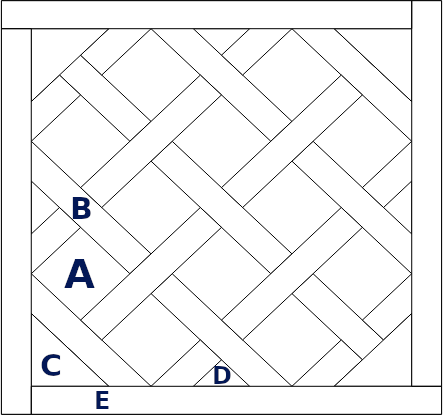<< Go back to Tech

# Versailles's Floor generator

Idea of the weekend.# Introduction

Versailles’s floor is a puzzle: many pieces to assemble together. I wanted to create a simple simulator for the most common pattern.

# Simulator

This is a simple javascript simulator, made with Bokeh.

You can play with using two sliders:

• The R slider modifies the trade-off between the square and the lines (of lenght a and b respectively).
• The length slider doesn’t change anything visual, this is only if you don’t want to compute the size of the pieces yourself. The length represents the total length (borders included).
Bokeh Plot

# Formulas

We will first forget about the total length. The reference unit will be R, the ratio between the large squares, and the spacing rectangles.## Main Components

Edge of A:

$a = R$

Width of B:

$b = 1 - R$

from which you get the diagonal:

$D_a = \sqrt{2 \times a^2}$ $D_b = \sqrt{2 \times b^2}$

For the rectangle B, $$D_b$$ is not the diagonal: it is the length of the smallest edge cut with an angle of 45°.

## Triangles

Diagonal of the angle’s triangle C:

$x = 2 \times a + b$

From which you get the side of the triangle:

$y = \sqrt{\frac{x^2}{2}}$

For the small triangle D, the hypotenuse is:

$z = D_a - D_b$

## Internal Length

The internal length (not the total one) is:

$L = 2 \times y + 4 \times D_b + z$

This is the length of a border E (and $$b$$ is the width).

## Converting Units

If we want to move from our unit defined as the length of two main elements glued together to the total size of a pannel, this is easy.

• The total length in our unit is $$L + 2 \times b$$
• The “true” total length is $$L_0$$

To convert all the previous numbers into the target unit, we just need to compute:

$w' = w \times \frac{L_0}{L + 2 \times b}$

where $$w$$ is the length to convert and $$w'$$ the resulting value.

# How to Generate that ?

Well, you need to find the coordinate of B rectangles.

Next, the simplest is to perform translation (horizontal and vertical) of $$D_a + D_b$$.

There are rectangles that are cut at 45°. To avoid finding the coordinates, the simplest thing to do (from a computer science perspective) is to add above these B rectangles the borders E. So they hide the edges.

>> You can subscribe to my mailing list here for a monthly update. <<